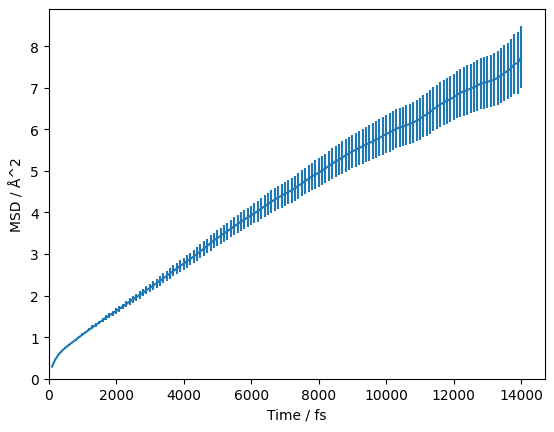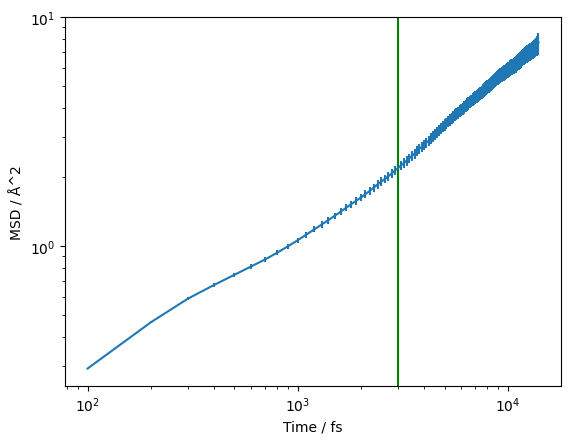# Diffusion coefficient from a VASP file#

Previously, we looked at obtaining accurate estimates for the mean-squared displacement with kinisi. Here, we show using the same class to evaluate the diffusion coefficient, using the kinisi methodology.

:

import numpy as np
from kinisi.analyze import DiffusionAnalyzer
from pymatgen.io.vasp import Xdatcar
np.random.seed(42)


There the p_params dictionary describes details about the simulation, and are documented in the parser module.

:

p_params = {'specie': 'Li',
'time_step': 2.0,
'step_skip': 50,
'min_obs': 50}


While the b_params dictionary describes the details of the bootstrapping process, these are documented in the diffusion module. Here, we indicate that we only want to investigate diffusion in the xy-plane of the system.

:

b_params = {'dimension': 'xy'}

:

xd = Xdatcar('./example_XDATCAR.gz')
diff = DiffusionAnalyzer.from_Xdatcar(xd, parser_params=p_params, bootstrap_params=b_params)

Reading Trajectory: 100%|██████████| 140/140 [00:00<00:00, 3282.81it/s]
Getting Displacements: 100%|██████████| 139/139 [00:00<00:00, 19205.07it/s]
Bootstrapping Displacements:  70%|██████▉   | 97/139 [00:13<00:03, 11.85it/s]/home/docs/checkouts/readthedocs.org/user_builds/kinisi/envs/latest/lib/python3.7/site-packages/kinisi-0.3.11-py3.7.egg/kinisi/diffusion.py:244: UserWarning: The maximum number of resamples has been reached, and the distribution is not yet normal.
warnings.warn("The maximum number of resamples has been reached, and the distribution is not yet normal.")
Bootstrapping Displacements: 100%|██████████| 139/139 [00:19<00:00,  7.02it/s]


In the above cells, we parse and determine the uncertainty on the mean-squared displacement as a function of the timestep. We should visualise this, to check that we are observing diffusion in our material and to determine the timescale at which this diffusion begins.

:

import matplotlib.pyplot as plt

:

plt.errorbar(diff.dt, diff.msd, diff.msd_std)
plt.axvline(diff.ngp_max, color='g')
plt.ylabel('MSD/Å$^2$')
plt.xlabel('$\Delta t$/ps')
plt.show()The vertical green line indicates the start of the diffusive regime, based on the maximum of the non-Gaussian parameter. We can also visualise this on a log-log scale, which helps to reveal the diffusive regime.

:

plt.errorbar(diff.dt, diff.msd, diff.msd_std)
plt.axvline(diff.ngp_max, color='g')
plt.ylabel('MSD/Å$^2$')
plt.xlabel('$\Delta t$/ps')
plt.yscale('log')
plt.xscale('log')
plt.show()It appears that the non-Gaussian parameter does an okay job at predicting the start of the diffusive regime for this system, so we will set use_ngp to True such that this is used in the fitting. If we wanted to use a different estimate, say 4 ps which looks more like the real start, we could use the keyword argument of dt_skip=4. The d_params dictionary defines parameters about the diffusion estimation, documented in the diffusion module.

:

d_params = {'use_ngp': True}


We can then run the diffusion analysis as follows.

:

diff.diffusion(d_params)

/home/docs/checkouts/readthedocs.org/user_builds/kinisi/envs/latest/lib/python3.7/site-packages/scipy/optimize/_numdiff.py:557: RuntimeWarning: invalid value encountered in subtract
df = fun(x) - f0
Likelihood Sampling: 100%|██████████| 1500/1500 [00:04<00:00, 303.95it/s]


This method estimates the correlation matrix between the timesteps and uses likelihood sampling to find the diffusion coefficient, $$D$$ and intercept (the units are cm :sup:2 /s and Å :sup:2 respectively). Now we can probe these objects.

We can get the median and 95 % confidence interval using,

:

diff.D, diff.intercept

---------------------------------------------------------------------------
TypeError                                 Traceback (most recent call last)
~/checkouts/readthedocs.org/user_builds/kinisi/envs/latest/lib/python3.7/site-packages/IPython/core/formatters.py in __call__(self, obj)
700                 type_pprinters=self.type_printers,
701                 deferred_pprinters=self.deferred_printers)
--> 702             printer.pretty(obj)
703             printer.flush()
704             return stream.getvalue()

~/checkouts/readthedocs.org/user_builds/kinisi/envs/latest/lib/python3.7/site-packages/IPython/lib/pretty.py in pretty(self, obj)
375                 if cls in self.type_pprinters:
376                     # printer registered in self.type_pprinters
--> 377                     return self.type_pprinters[cls](obj, self, cycle)
378                 else:
379                     # deferred printer

~/checkouts/readthedocs.org/user_builds/kinisi/envs/latest/lib/python3.7/site-packages/IPython/lib/pretty.py in inner(obj, p, cycle)
553                 p.text(',')
554                 p.breakable()
--> 555             p.pretty(x)
556         if len(obj) == 1 and type(obj) is tuple:
557             # Special case for 1-item tuples.

~/checkouts/readthedocs.org/user_builds/kinisi/envs/latest/lib/python3.7/site-packages/IPython/lib/pretty.py in pretty(self, obj)
392                         if cls is not object \
393                                 and callable(cls.__dict__.get('__repr__')):
--> 394                             return _repr_pprint(obj, self, cycle)
395
396             return _default_pprint(obj, self, cycle)

~/checkouts/readthedocs.org/user_builds/kinisi/envs/latest/lib/python3.7/site-packages/IPython/lib/pretty.py in _repr_pprint(obj, p, cycle)
698     """A pprint that just redirects to the normal repr function."""
699     # Find newlines and replace them with p.break_()
--> 700     output = repr(obj)
701     lines = output.splitlines()
702     with p.group():

TypeError: __repr__ returned non-string (type numpy.ndarray)


Or we can get all of the sampled values from one of these objects.

:

diff.D.samples

:

array([1.44940360e-05, 1.38992901e-05, 1.49257699e-05, ...,
1.46193283e-05, 1.54353138e-05, 1.45811571e-05])


Having completed the analysis, we can save the object for use later (such as downstream by a plotting script).

:

diff.save('example.hdf')


The data is saved in a HDF5 file format which helps us efficiently store our data. We can then load the data with the following class method.

:

loaded_diff = DiffusionAnalyzer.load('example.hdf')


We can plot the data with the credible intervals from the $$D$$ and $$D_{\text{offset}}$$ distribution.

:

credible_intervals = [[16, 84], [2.5, 97.5], [0.15, 99.85]]
alpha = [0.6, 0.4, 0.2]

for i, ci in enumerate(credible_intervals):
alpha=alpha[i],
color='#0173B2',
lw=0)
plt.ylabel('MSD/Å$^2$')
plt.xlabel('$\Delta t$/ps')
plt.show()Additionally, we can visualise the distribution of the diffusion coefficient that has been determined.

:

plt.hist(loaded_diff.D.samples, density=True)
plt.xlabel('$D$/cm$^2$s$^{-1}$')
plt.ylabel('$p(D$/cm$^2$s$^{-1})$')
plt.show()Or the joint probability distribution for the diffusion coefficient and intercept.

:

from corner import corner

:

corner(loaded_diff.flatchain, labels=['$D$/cm$^2$s$^{-1}$', 'intercept/Å$^2$'])
plt.show()